# Maximum Xor Queries | Trie

In this article, we will solve the most asked interview question: “Maximum Xor Queries”.

Problem LinkMaximum Xor Queries

Problem Statement:

You are given an array/list ‘ARR’ consisting of ‘N’ non-negative integers. You are also given a list ‘QUERIES’ consisting of ‘M’ queries, where the ‘i-th’ query is a list/array of two non-negative integers ‘Xi’, ‘Ai’, i.e ‘QUERIES[i]’ = [‘Xi’, ‘Ai’].

The answer to the ith query, i.e ‘QUERIES[i]’ is the maximum bitwise xor value of ‘Xi’ with any integer less than or equal to ‘Ai’ in ‘ARR’.

You should return an array/list consisting of ‘N’ integers where the ‘i-th’ integer is the answer of ‘QUERIES[i]’.

Examples:

```Example 1:
Input:

2
5 2
0 1 2 3 4
1 3
5 6
1 1
1
1 0

Output:
3 7
-1

Explanation:
In the first test case, the answer of query [1, 3] is 3 because 1^2 = 3 and 2 <= 3,  and the answer of query [5, 6] is 7 because  5 ^ 2 = 7 and 2 <= 6.

In the second test case, no element is less than or equal to 0 in the given array ‘ARR’.

Example 2:
Input:
2
6 3
6 6 3 5 2 4
6 3
8 1
12 4
5 2
0 0 0 0 0
1 0
1 1

Output:
5 -1 15
1 1
```

## Solution:

Disclaimer: Don’t jump directly to the solution, try it out yourself first.

## Solution 1 : Brute-Force

Approach:

• For every query q[i] = [xi,ai], initialize maxXOR = 0.
• Create a vector/list(ans) to store the answers for each of the query.
• Now run a for loop (j – > (0,n-1) on the array and check if (ar[j] <= ai) then calculate XOR value for(xi,ar[j]), if(maxXOR < (xi^ar[j]) then update maxXOR as (xi^ar[j]).
• After coming out of the for loop store this maxXOR into the array/list(ans).
• Finally, return the vector/list(ans).

Code:

## C++ Code

``````#include<bits/stdc++.h>

using namespace std;

vector < int > maxXorQueries(vector < int > & arr, vector < vector
< int >> & queries) {
int n = arr.size();
int m = queries.size();
vector < int > ans(m, -1);
for (int i = 0; i < m; i++) {
for (int j = 0; j < n; j++) {
if (arr[j] <= queries[i]) {
ans[i] = max(ans[i], arr[j] ^ queries[i]);
}
}
}
return ans;
}
int main() {
int t;
cin >> t;
while (t--) {
int n, m;
cin >> n >> m;
vector < int > arr(n);
for (int i = 0; i < n; i++) {
cin >> arr[i];
}
vector < vector < int >> queries;

for (int i = 0; i < m; i++) {
vector < int > temp;
int xi, ai;
cin >> xi >> ai;
temp.push_back(xi);
temp.push_back(ai);
queries.push_back(temp);
}

vector < int > ans = maxXorQueries(arr, queries);
for (int j = 0; j < ans.size(); j++) {
cout << ans[j] << " ";
}
cout << endl;
}

}``````

Input:

2
5 2
0 1 2 3 4
1 3
5 6
1 1
1
1 0

Output:

3 7
-1

Time Complexity: O(Q * N)

Reason: for every query, we are running a for loop on N elements and calculating xor value. So for Q queries, it will be (Q * N).

Space Complexity: O(1)

## Java Code

``````import java.util.*;
class Solution {
public static ArrayList < Integer > maxXorQueries(ArrayList < Integer > arr,
ArrayList < ArrayList < Integer >> queries) {
int n = arr.size();
int m = queries.size();
ArrayList < Integer > ans = new ArrayList < > (m);
for (int i = 0; i < m; i++) {
}
for (int i = 0; i < m; i++) {
for (int j = 0; j < n; j++) {
if (arr.get(j) <= queries.get(i).get(1)) {
int temp = Math.max(ans.get(i), arr.get(j) ^ queries.get(i).get(0));
ans.set(i, temp);
}
}
}
return ans;
}
}
public class Main {

public static void main(String[] args) {
Scanner sc = new Scanner(System.in);
int t;
t = sc.nextInt();
for (int j = 0; j < t; j++) {
int n, m;
n = sc.nextInt();
m = sc.nextInt();
ArrayList < Integer > arr = new ArrayList < Integer > (n);
for (int i = 0; i < n; i++) {
}

ArrayList < ArrayList < Integer >> queries = new ArrayList < ArrayList
< Integer > > (m);

for (int i = 0; i < m; i++) {
ArrayList < Integer > temp = new ArrayList < Integer > (2);
}

Solution s = new Solution();

ArrayList < Integer > ans = new ArrayList < Integer > (n);
ans = s.maxXorQueries(arr, queries);

for (int it: ans) {
System.out.print(it + " ");
}
System.out.print('\n');
}

}
}``````

Input:

2
5 2
0 1 2 3 4
1 3
5 6
1 1
1
1 0

Output:

3 7
-1

Time Complexity: O(Q * N)

Reason: for every query, we are running a for loop on N elements and calculating xor value. So for Q queries, it will be (Q * N).

Space Complexity: O(1)

## Solution 2: Using Trie Data Structure

Prerequisite: L6. Maximum XOR of Two Numbers in an Array

1. This problem is an extension of the previous problem.In order to understand this problem you should have done the previous problem which is Maximum XOR of Two Numbers in an Array.

2. We know how to get maxXor value for an element x and array/list (arr). Now, Unlike the previous problem the array/list (arr) should contain only the elements which are less than or equal to ai.

3. Firstly, sort the array/list(arr), and for each query create a trie and push all the elements which are less than or equal to ai, and calculate getMax.

Do we really need to create a new trie for every query ??

No, since we are only working on a single array/list. Let’s try a different way to solve this.

Let’s solve it by using the offlineQueries.

OfflineQueries:

• Create a vector/ArrayList of type (int,pair<int,int>).//(ai,{xi,i})
• Suppose the given array/list[] = {1,2,3,4,5}
• Queries as given below:
• offlineQueries vector/list would look like as below
• Sort the offlineQueries based on the ai value, After sorting the offlineQueries would be as follows
• Keep a ptr = 0 pointing to the start of the array/list.
• Now insert all the elements which are less than or equal to ai.
• If we are inserting an element then move the ptr by 1.
• Finally, Calculate getMax(xi) and store the calculated value in it’s queryIdx.

Dry-Run:

• Query – > [1, [3, 3]]

Here ai = 1,xi = 3,queryIdx = 3,ptr = 0.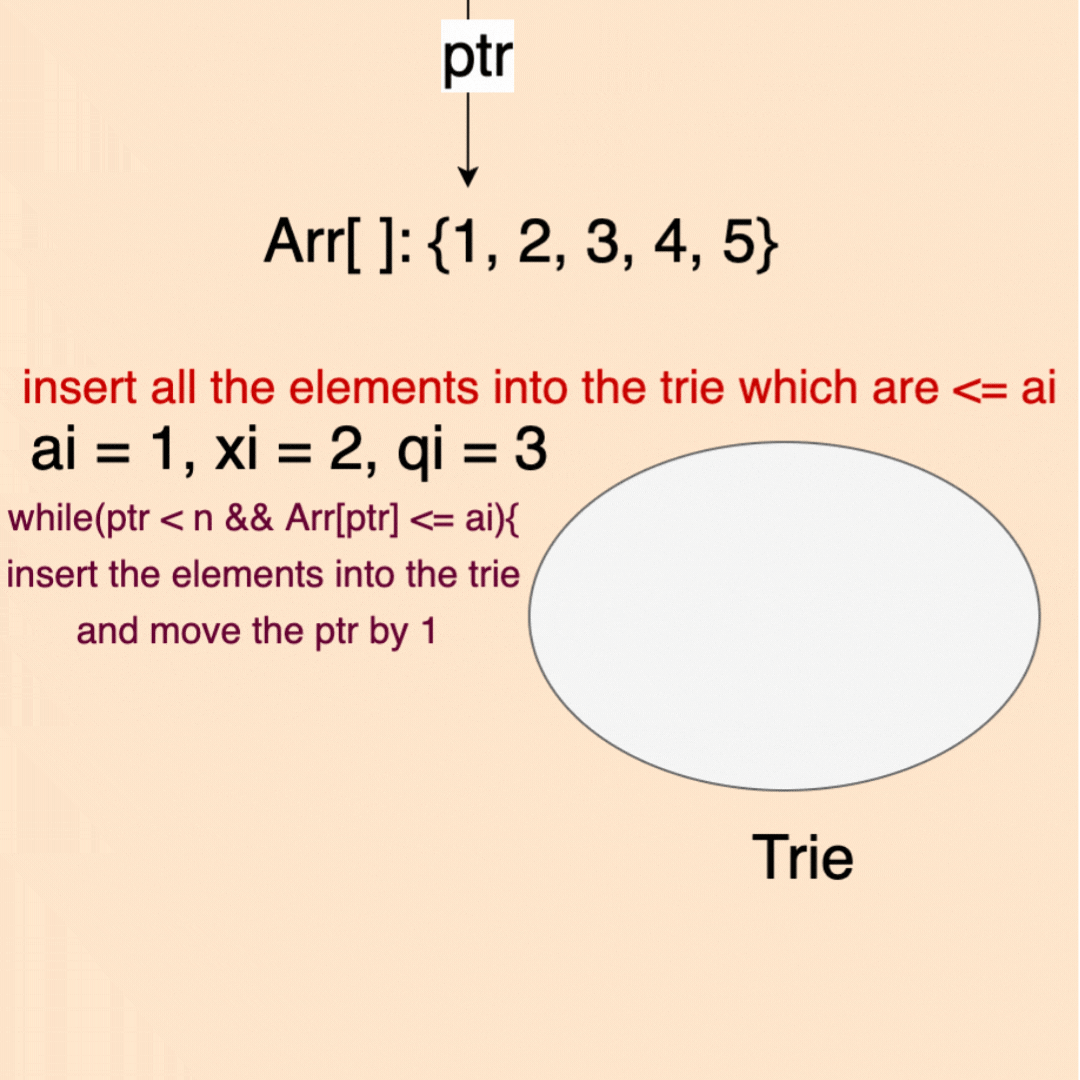• Query – > [2, [5, 1]]

Here ai = 2,xi = 5,queryIdx = 3,ptr = 1.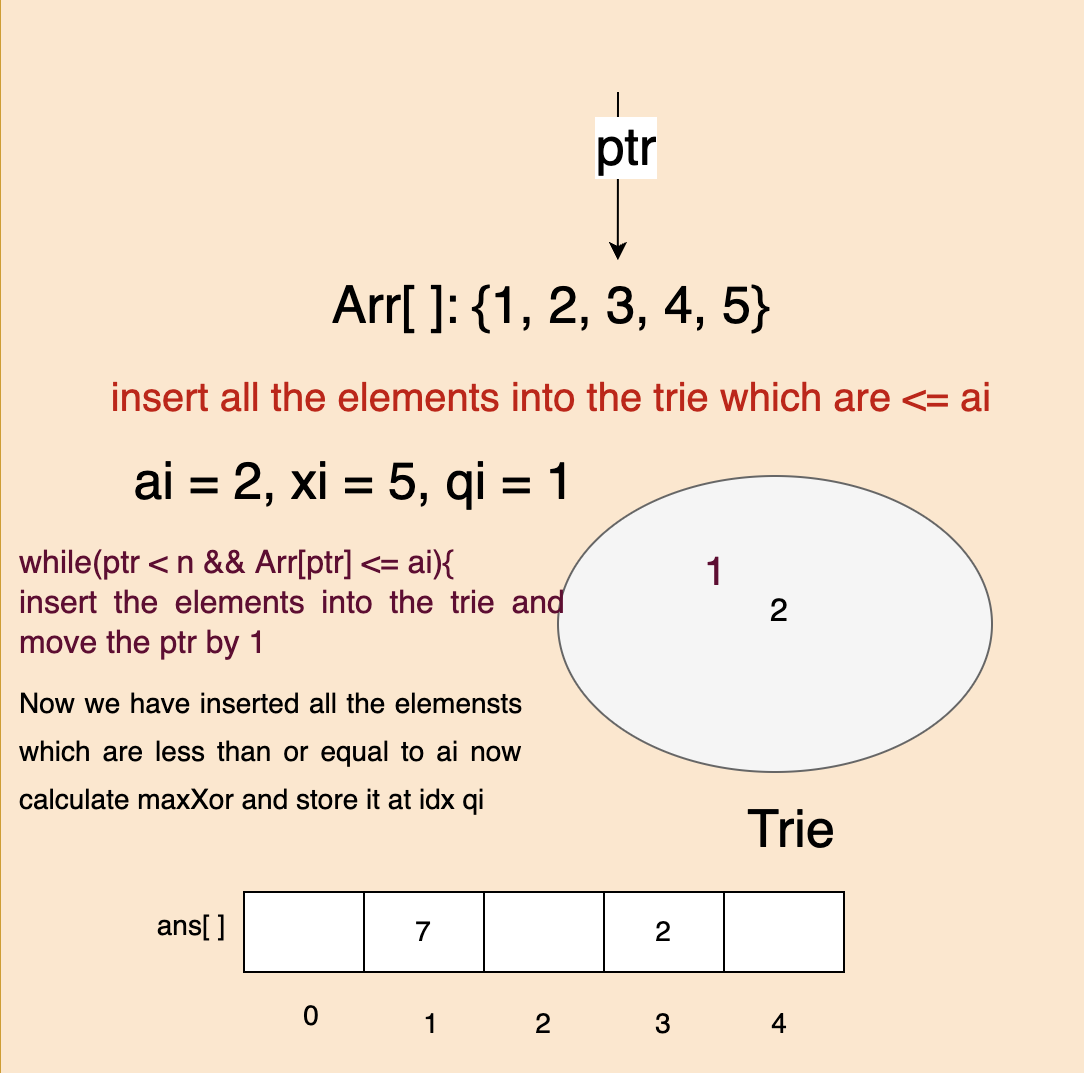• Query – > [4, [3, 0]]

Here ai = 4,xi = 4,queryIdx = 0,ptr = 2.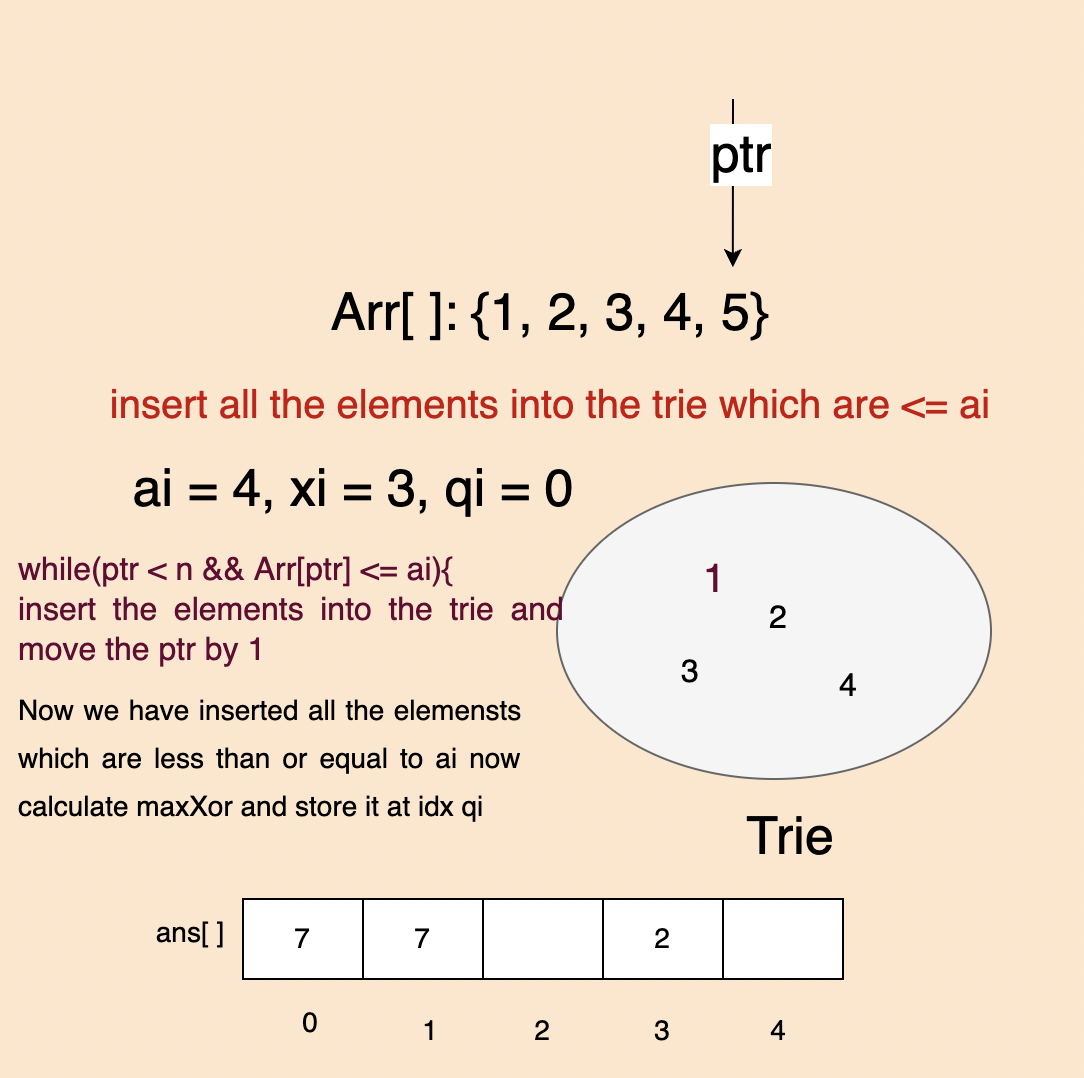• Query – > [5, [2, 2]]

Here ai = 5,xi = 2,queryIdx = 2,ptr = 4.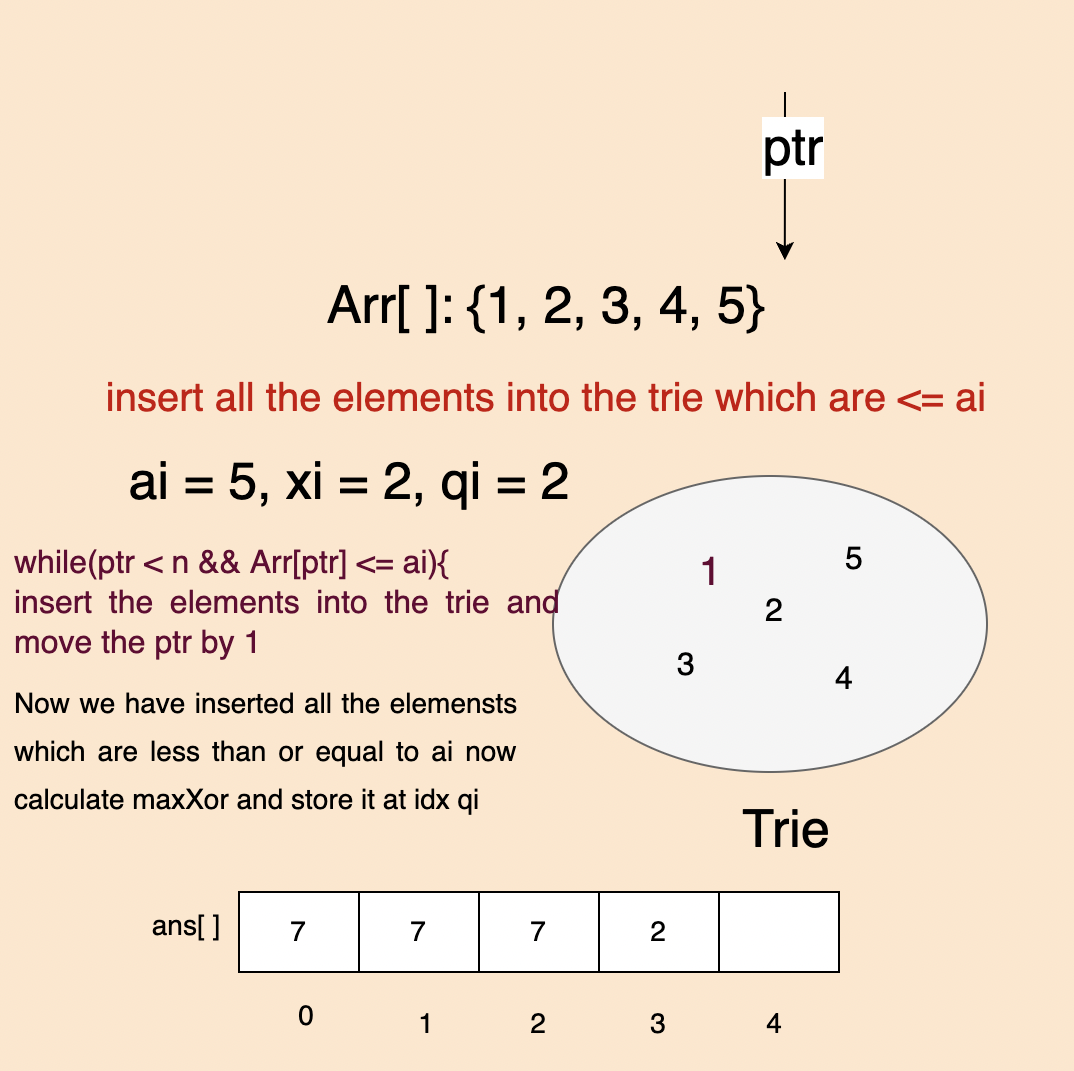• Query – > [6, [6, 4]]

Here ai = 6,xi = 6,queryIdx = 4,ptr = 6.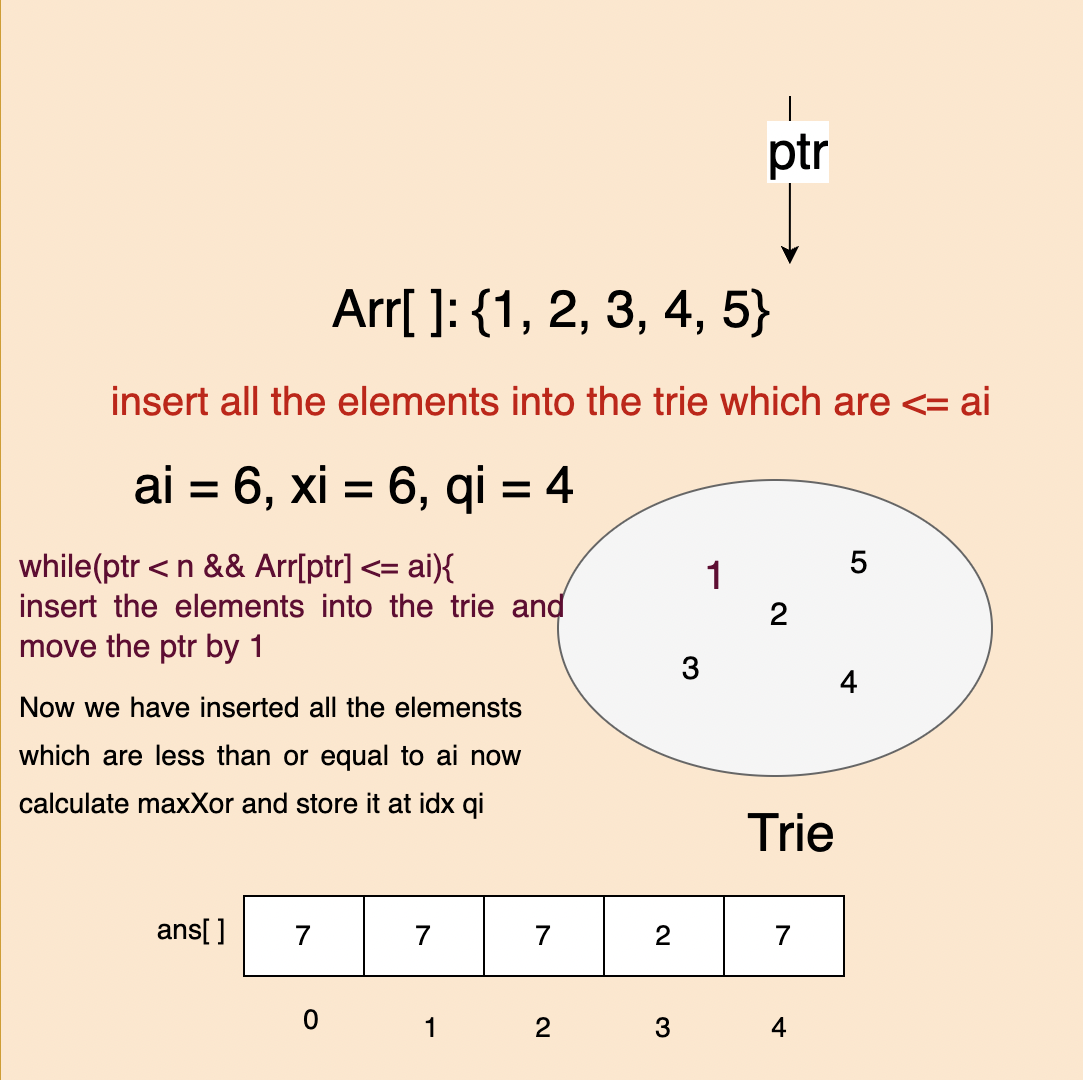### Is this an efficient way to solve the problem?

Yes, Instead of creating a trie for every query we just sorted the query based on ai, and calculated the maxXor for each query, and stored the result in its queryIdx.

Note: From the above approach, we can see that for each query we are not removing any elements from trie instead we may add the new elements to the trie or may not.

Code:

## C++ Code

``````#include<bits/stdc++.h>

using namespace std;

struct Node {

bool containsKey(int ind) {
return (links[ind] != NULL);
}
Node * get(int ind) {
}
void put(int ind, Node * node) {
}
};
class Trie {
private: Node * root;
public:
Trie() {
root = new Node();
}

public:
void insert(int num) {
Node * node = root;
// cout << num << endl;
for (int i = 31; i >= 0; i--) {
int bit = (num >> i) & 1;
if (!node -> containsKey(bit)) {
node -> put(bit, new Node());
}
node = node -> get(bit);
}
}
public:
int findMax(int num) {
Node * node = root;
int maxNum = 0;
for (int i = 31; i >= 0; i--) {
int bit = (num >> i) & 1;
if (node -> containsKey(!bit)) {
maxNum = maxNum | (1 << i);
node = node -> get(!bit);
} else {
node = node -> get(bit);
}
}
return maxNum;
}
};
vector < int > maxXorQueries(vector < int > & arr, vector < vector < int
>> & queries) {
vector < int > ans(queries.size(), 0);
vector < pair < int, pair < int, int >>> offlineQueries;
sort(arr.begin(), arr.end());
int index = 0;
for (auto & it: queries) {
offlineQueries.push_back({
it,
{
it,
index++
}
});
}
sort(offlineQueries.begin(), offlineQueries.end());
int i = 0;
int n = arr.size();
Trie trie;

for (auto & it: offlineQueries) {
while (i < n && arr[i] <= it.first) {
trie.insert(arr[i]);
i++;
}
if (i != 0) ans[it.second.second] = trie.findMax(it.second.first);
else ans[it.second.second] = -1;
}
return ans;
}
int main() {
int t;
cin >> t;
while (t--) {
int n, m;
cin >> n >> m;
vector < int > arr(n);
for (int i = 0; i < n; i++) {
cin >> arr[i];
}
vector < vector < int >> queries;

for (int i = 0; i < m; i++) {
vector < int > temp;
int xi, ai;
cin >> xi >> ai;
temp.push_back(xi);
temp.push_back(ai);
queries.push_back(temp);
}

vector < int > ans = maxXorQueries(arr, queries);
for (int j = 0; j < ans.size(); j++) {
cout << ans[j] << " ";
}
cout << endl;
}

}``````

Input:

2
5 2
0 1 2 3 4
1 3
5 6
1 1
1
1 0

Output:

3 7
-1

Time Complexity:O(M) + O(MlogM) + O(M*32 + N*32)

Space Complexity:O(32*N)

Reason: O(M) for creating a Vector/ArrayList of OfflineQueries, O(MlogM) for sorting the offlineQueries,O(M*32 + N*32) for inserting the elements into a trie and calculating the maxXor value.//32 since we are storing the integer in 32 bit format.

## Java Code

``````import java.util.ArrayList;
import java.util.*;
class Node {

Node links[] = new Node;

public Node() {}
boolean containsKey(int ind) {
return (links[ind] != null);
}
Node get(int ind) {
}
void put(int ind, Node node) {
}
};
class Trie {
private static Node root;

//Initialize your data structure here

Trie() {
root = new Node();
}

//Inserts a word into the trie

public static void insert(int num) {
Node node = root;
for (int i = 31; i >= 0; i--) {
int bit = (num >> i) & 1;
if (!node.containsKey(bit)) {
node.put(bit, new Node());
}
node = node.get(bit);
}
}

public int getMax(int num) {
Node node = root;
int maxNum = 0;
for (int i = 31; i >= 0; i--) {
int bit = (num >> i) & 1;
if (node.containsKey(1 - bit)) {
maxNum = maxNum | (1 << i);
node = node.get(1 - bit);
} else {
node = node.get(bit);
}
}
return maxNum;
}
};
class Solution {
public static ArrayList < Integer > maxXorQueries(ArrayList < Integer > arr,
ArrayList < ArrayList < Integer >> queries) {
Collections.sort(arr);
ArrayList < ArrayList < Integer >> offlineQueries = new ArrayList < ArrayList
< Integer >> ();
int m = queries.size();
for (int i = 0; i < m; i++) {
ArrayList < Integer > temp = new ArrayList < Integer > ();
}
Collections.sort(offlineQueries, new Comparator < ArrayList < Integer >> () {
@Override
public int compare(ArrayList < Integer > a, ArrayList < Integer > b) {
return a.get(0).compareTo(b.get(0));
}
});
int ind = 0;
int n = arr.size();
Trie trie = new Trie();
ArrayList < Integer > ans = new ArrayList < Integer > (m);
for (int i = 0; i < m; i++) ans.add(-1);
for (int i = 0; i < m; i++) {
while (ind < n && arr.get(ind) <= offlineQueries.get(i).get(0)) {
trie.insert(arr.get(ind));
ind++;
}
int queryInd = offlineQueries.get(i).get(2);
if (ind != 0) ans.set(queryInd, trie.getMax(offlineQueries.get(i).get(1)));
else ans.set(queryInd, -1);
}
return ans;
}
}
public class Main {
public static void main(String[] args) {
Scanner sc = new Scanner(System.in);
int t;
t = sc.nextInt();
for (int j = 0; j < t; j++) {
int n, m;
n = sc.nextInt();
m = sc.nextInt();
ArrayList < Integer > arr = new ArrayList < Integer > (n);
for (int i = 0; i < n; i++) {
}

ArrayList < ArrayList < Integer >> queries = new ArrayList < ArrayList <
Integer > > (m);

for (int i = 0; i < m; i++) {
ArrayList < Integer > temp = new ArrayList < Integer > (2);
}

Solution s = new Solution();

ArrayList < Integer > ans = new ArrayList < Integer > (n);
ans = s.maxXorQueries(arr, queries);

for (int it: ans) {
System.out.print(it + " ");
}
System.out.print('\n');
}
}
}
``````

Input:

2
5 2
0 1 2 3 4
1 3
5 6
1 1
1
1 0

Output:

3 7
-1

Time Complexity:O(M) + O(MlogM) + O(M*32 + N*32)

Space Complexity:O(32*N)

Reason: O(M) for creating a Vector/ArrayList of OfflineQueries, O(MlogM) for sorting the offlineQueries, O(M*32 + N*32) for inserting the elements into a trie and calculating the maxXor value.//32 since we are storing the integer in 32-bit format.

Special thanks to P.C.Bhuvaneshwari for contributing to this article on takeUforward. If you also wish to share your knowledge with the takeUforward fam, please check out this article